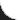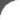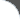Home | Tutorials | Articles | Videos | Products | Tools | Search
 Interviews | Open Source | Tag Cloud | Follow Us | Bookmark | ContactIn Browser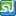StumbleUpon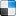del.icio.us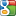Google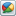Google Buzz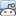reddit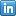LinkedIn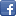Facebook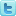TwitterLinkedin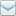E-Mail
 Excel > JExcel API > How to add Min Max Formula to Excel Spreadsheet

Java Excel API is an open source java library to read, write and modify Excel spread sheets. This requires the library jxl-2.6.12.jar to be in classpath. The following example shows how to add min&max formula to a Cell in Excel Spreadsheet.

 File Name  : com/bethecoder/tutorials/jexcelapi/write/MinMaxFormulaTest.java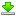Author  : Sudhakar KV Email  : [email protected]

 ``` package com.bethecoder.tutorials.jexcelapi.write; import java.io.File; import java.io.IOException; import jxl.Workbook; import jxl.read.biff.BiffException; import jxl.write.Formula; import jxl.write.Label; import jxl.write.Number; import jxl.write.WritableSheet; import jxl.write.WritableWorkbook; import jxl.write.WriteException; import jxl.write.biff.RowsExceededException; public class MinMaxFormulaTest {   /**    * @param args    * @throws IOException     * @throws IOException     * @throws WriteException     * @throws BiffException     */   public static void main(String[] args) throws IOException, WriteException {     //Creates a writable workbook with the given file name     WritableWorkbook workbook = Workbook.createWorkbook(new File("C:/JXL/MinMaxFormula.xls"));     WritableSheet sheet = workbook.createSheet("My Sheet", 0);     Number numCell = new Number(1, 0, 10);     sheet.addCell(numCell);     Number numCell2 = new Number(1, 1, 20);     sheet.addCell(numCell2);          Number numCell3 = new Number(1, 2, 30);     sheet.addCell(numCell3);          Number numCell4 = new Number(1, 3, 40);     sheet.addCell(numCell4);          addMinMaxCells(sheet, "MIN(B1:B4)", 4);     addMinMaxCells(sheet, "MAX(B1:B4)", 5);     addMinMaxCells(sheet, "MIN(6, 2, 1, 0, 8, 9)", 6);     addMinMaxCells(sheet, "MAX(6, 2, 1, 0, 8, 9)", 7);          //Writes out the data held in this workbook in Excel format     workbook.write();      //Close and free allocated memory      workbook.close();    }   private static void addMinMaxCells(       WritableSheet sheet,        String formula, int row) throws RowsExceededException, WriteException {          //Create a formula for average     Formula formulaCell = new Formula(1, row, formula);     sheet.addCell(formulaCell);          //Create label for average     Label formulaLabel = new Label(0, row, formula);     sheet.addCell(formulaLabel);   } }```

It gives the following output,